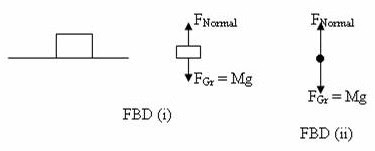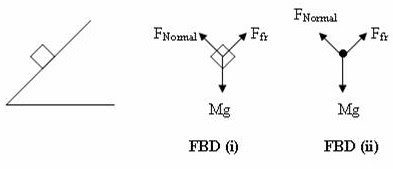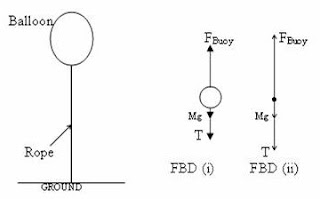## Pages

`“Life is like riding a bicycle.  To keep your balance you must keep moving.”–Albert Einstein`

## Thursday, February 17, 2011

### Free Body Diagrams for AP Physics B and C

“To give pleasure to a single heart by a single act is better than a thousand heads bowing in prayer”

– Mahatma Gandhi

As AP Physics B and C aspirants you need to master the technique of drawing free body diagrams (FBD) without mistakes. You might have encountered many situations, especially in mechanics, where you were required to draw correct free body diagrams. Drawing free body diagrams at the level expected of you is ordinarily simple. Yet there may be many among you who have genuine doubts in this area.

A free body diagram of an object is one in which all the forces acting on the object are pictorially represented. The object may be the part of a complex system. But in the free body diagram of an object, you need to show the object alone (represented by a simple geometrical figure such as a dot or a small square or a small rectangle or the outline of the object) along with the forces represented by directed line segments (arrows). The length of the arrows, as usual in vector representation, should represent the magnitude of the force while the direction of the arrow should indicate the direction of the force. Since the free body diagram is a pictorial representation of the object along with the forces acting on it, used for simplifying your analysis of a problem, you need not worry about the exact length of the arrow and its exact direction. But the labeling of the magnitude and direction of the known forces should be correct.

In AP Physics free response questions often you may be asked to represent an object (such as a skier moving along a ramp or a body floating on the surface of a liquid) by a dot and to draw and label the forces that act on the object. Understand that you are being prompted to draw a simple free body diagram.

We will now draw the free body diagrams of some typical objects and systems in typical situations so that you will become more confident for facing your AP Physics Exam.

(1) A box of mass M at rest on a horizontal table:The box can be represented by a small rectangle in the free body diagram as shown in FBD (i) in the figure. The forces acting on the box are the gravitational pull (weight) FGr and the normal force FNormal exerted by the surface of the table. These are of equal magnitude, but opposite in direction (FGr being downwards and FNormal upwards. Surely, FGr = Mg.

[Even if you dispense with the symbol FGr for the gravitational pull and just write the weight Mg as label for it, your free body diagram will be alright].

If you were asked to represent the box by a dot, you would draw the free body diagram as shown in FBD (ii).

You know that the weight of the box acts through the centre of gravity of the box where as the normal force is exerted at the contact surface. You can draw the force vectors taking these into consideration; but there is no harm in showing them as in FBD (i) since the body has neither translational motion nor rotational motion since the equal and opposite forces have their lines of action coinciding.

When you are asked to represent the object by a dot, the question setter is in fact making things simpler for you.

(2) A block of mass M at rest on an incline:

In this case three forces act on the object. They are:

(i) The weight Mg of the block

(ii) The normal force FNormal exerted by the incline and

(iii) The force of friction Ffr preventing the block from sliding downThe object is represented by a small rectangle in the free body diagram shown in FBD (i) in the figure. The frictional force Ffr acts along the surface of the block while the weight of the block acts through the centre of gravity of the block. The normal force in this case is opposite to the component of the weight normal to the inclined surface. Since the block does not rotate, the three forces acting on it have to be concurrent and so you need not be confused as to how to show the lines of action of the three forces. If you are asked to represent the block by a dot as shown in FBD (ii) you will be relieved of confusion.

[Note that the component of weight normal to the inclined surface is Mg cosθ where θ is the inclination of the plane (to the horizontal) and hence the magnitude of the normal force FNormal is Mg cosθ. The frictional force Ffr is equal and opposite to the component (Mg sinθ) of the weight (of the block) parallel to the incline, as long as the block is at rest. If the block is just beginning to slide down, Ffr = μs Mg cosθ where μs is the coefficient of limiting friction (coefficient of static friction). If the block is in motion, Ffr = μk Mg cosθ where μk is the coefficient of kinetic friction. You need not show these details in the free body diagram. But you need to know these things in solving problems].

(3) A block of mass M sliding down an incline under gravity (No other external force applied):

The free body diagram will be the same as that shown in (2) above. But in this case the three forces are not balanced and so you have to show a smaller magnitude for the frictional force Ffr so that there is a net force acting down the plane to move the block

If you are asked take into account the viscous drag force FD due to air, you have to show it along the direction of Ffr.

(4) A toy boat of mass m1 carrying pebbles of mass m2 floats on the surface of water in a jar:

In this case the object is the toy boat carrying glass beads. The total mass of the object is m1 + m2. Two forces act on the object. They are:

(i) The weight (m1 + m2)g of the object acting vertically downwards

(ii) The force of buoyancy FBuoy acting vertically upwards.

You may represent the object by a small rectangle and indicate the weight and the force of buoyancy respectively by downward and upward arrows of equal length as indicated in FBD (i). Since the floating object is in equilibrium, the two vectors have to be equal and opposite and their lines of action must coincide.

[The weight acts through the centre of gravity of the object where as the force of buoyancy acts through the centre of buoyancy (centre of gravity of the displaced liquid). But you may ignore such details].

If you represent the object by a dot, things become quite simple and you get the free body diagram as shown in FBD (ii).

(5) An iron block of mass M suspended (from a rigid support) using a string and partially (or fully) submerged in a liquid.

In this case three forces act on the object (iron block). They are:

(i) The real weight Mg of the block acting vertically downwards

(ii) The force of buoyancy FBuoy acting vertically upwards

(iii) The tension T in the string, acting vertically upwards

The free body diagram of the iron block can be drawn either as in FBD (i) or as in FBD (ii).

Since the iron block is in equilibrium, you have to draw the magnitudes of the forces such that magnitude of Mg = Magnitude of (FBuoy + T).

[Free body diagrams you draw in your answer sheets are rough sketches and hence no examiner will expect you to show the exact magnitudes of the forces].

(6) A skier of mass M skiing down a frictionless incline with a drag force Fd due to air opposing her motion

Three forces act on the object (skier). They are:

(i) The weight Mg of the skier acting vertically downwards

(ii) The drag force FD acting parallel to and up the incline

(ii) The normal force FNormal exerted by the incline.Usually you will be asked to represent the skier by a dot. You will draw the free body diagram of the skier as shown.

[If there is friction along the incline, you will have to show the frictional force also in same direction as that of the drag force].

(7) A cricket ball of mass m moving through air after being hit by a batsman (with negligible air resistance)

Since air resistance is negligible, the only force acting on the ball is its weight mg acting vertically downwards. The free body diagram is therefore very simple as shown in the adjoining figure.

[The direction of motion of the ball is immaterial in this simple case of negligible air resistance and you get the same free body diagram at all positions of the ball along its trajectory in air. But, if air resistance (drag force due to air) FD is significant and is to be considered, you will have to show it directed opposite to the direction of motion of the ball. This direction will change continuously with time if the ball is following a parabolic path in air. If you are asked to draw the free body diagram at the instant the ball is at the highest point of its parabolic trajectory, the drag force will be horizontal and opposite to the direction of motion of the ball. But if the ball is projected vertically up, it will be momentarily at rest at the highest point and hence the viscous drag force will be zero there].

(8) Blocks A and B of masses M1 and M2 (M1 > M2) tied to the ends of a string (of negligible mass) passing over a frictionless fixed pulley, with block A resting on the ground and block B hanging in air

You are required to draw the free body diagrams of block A as well as block B.

The arrangement of the blocks and pulley is shown in the figure. The required free body diagrams are also shown.

Three forces act on block A. They are:

(i) The weight m1g of the block acting vertically downwards

(ii) The normal force FNormal exerted by the ground, acting vertically upwards

(iii) The tension T in the string, acting vertically upwards

The important thing to be noted in the case of block A is that the normal force FNormal exerted on it by the ground is not of magnitude M1g but is less than M1g. The value is (M1 M2) g and the tension T in the string is M2 g

Two forces act on block B. They are:

(i) The weight M1g of the block acting vertically downwards

(ii) The tension T in the string, acting vertically upwards

[Since the same segment of string connects the two masses, the tension in the string has the same value].

The above situation can be suitably modified in your question paper. For instance, the block A can be a man of mass 70 kg standing on the ground and holding the end of the string and the block B can be a boy of mass 30 kg suspended from the other end of the string!

[If both blocks A and B were in air, the system of masses will move with an acceleration since there is a net driving force (M1–M2)g acting on the system. The free body diagram of block A will be similar to that of block B and the normal force FNormal will be absent. The magnitude of the tension T in the string will be greater than M1g, but less than M1g].

(9) A tethered hydrogen balloon of mass M kept stationary in air. The mass of the rope is negligible.You are required to draw the free body diagram of the balloon.

Three forces act on the balloon. They are:

(i) The weight Mg of the balloon, acting vertically downwards.

(ii) The force of buoyancy FBuoy acting vertically upwards.

(iii) The tension T in the string, acting vertically downwards.

Since the mean density of the hydrogen balloon is less than that of air, the force of buoyancy will be greater than the weight of the balloon. The weight Mg of the balloon and the tension T in the rope together prevent the balloon from rising up.

The free body diagram of the balloon can be drawn as shown in FBD (i). If you were asked to represent the balloon by a dot, you would draw the free body diagram as shown in FBD (ii).

## Monday, February 7, 2011

### Nuclear Physics - Multiple Choice Practice Questions for AP Physics B

“Maturity is often more absurd than youth and very frequently is most unjust to youth”

– Thomas A. Edison

Essential points to be remembered in nuclear physics were discussed in the post dated 24th December 2008. A few multiple choice practice questions on nuclear physics were discussed in the post dated 30th December 2008, followed by a free response practice question in the post dated 13th January 2009. You can access all those posts by clicking on the label ‘nuclear physics’ below this post.

Today we will discuss a few more multiple choice practice questions in this section:

(1) The density of nuclear matter is

(a) directly proportional to the number protons in the nucleus

(b) directly proportional to the number neutrons in the nucleus

(c) directly proportional to the square of the number of nucleons in the nucleus

(d) directly proportional to the 4th power of the number of nucleons in the nucleus

(e) independent of the number nucleons in the nucleus

The correct option is (e). Nuclear radius R is given by

R =R0A1/3

where R0 = 1.2×10–15 m and A is the mass number (or, nucleon number).

The volume of the nucleus which is proportional to R3 is therefore proportional to the mass number A. Since the density is the ratio of mass to volume, it follows that the density of the nucleus is independent of the mass number and is constant (approximately 2.3×1017 kgm–3).

(2) Nuclear force is

(a) short range and charge dependent

(b) long range and charge dependent

(c) short range and charge independent

(d) long range and charge independent

(e) electromagnetic in nature

Nuclear force is a short range force produced by the exchange of π-mesons between the nucleons.

The force between a proton and a neutron is produced because of the exchange of charged π-mesons (π+ and π) where as the force between two protons and that between two neutrons is produced by the exchange of uncharged π-mesons (π0).

Nuclear force is a strong attractive force (in fact, the strongest natural force) and is charge independent.

The correct option is (c).

(3) In the nuclear reaction given by

7N14 + 2He4 = mXn + 1H1, what is the nucleus mXn?

(a) Oxygen of mass number 18

(b) Nitrogen of mass number 18

(c) Oxygen of mass number 16

(d) Oxygen of mass number 17

(e) Nitrogen of mass number 17

Since the total mass number on the left hand side of the equation is 18, the mass number of X has to be 17 (for balancing the equation).

[14 + 4 = n + 1 from which n = 17]

The atomic number of X (or, the number of protons in the nucleus X) is 8 since the total atomic number on the left hand side of the equation is 9.

[7 + 2 = m + 1 from which m = 8]

Therefore the nucleus X is that of oxygen (8O17)

(4) Cobalt 60 is a radioactive source with a half life of 5.27 years. You may take it as 5 years. After how many years will the activity of a sample of cobalt 60 be decreased to 1/16 its original activity?

(a) 10 years

(b) 16 years

(c) 20 years

(d) 32 years

(e) 40 years

The activity (number of disintegrations per second) is directly proportional to the number of undecayed nuclei in the sample. Since the number of undecayed nuclei reduces to half the initial value in every half life period, the activity also reduces to half the initial value in every half life period. Therefore, after 5 years the activity becomes half the initial value; after 10 years the activity becomes 1/4 the initial value; after 15 years it becomes 1/8 the initial value and after 20 years the activity becomes 1/16 the initial value. The correct option is (c).

[The activity A after n half lives is given by A = A0/2n where A0 is the initial activity. Therefore, 1/16 = 1/2n so that 2n = 16 from which n = 4. Four half lives = 20 years].

(5) In a typical fission reaction, a U235 nucleus absorbs a slow neutron and becomes a compound nucleus U236 in a highly excited state. U236 then undergoes fission, producing two fission fragments (Xe140 and Sr94) and two neutrons. Typically what should be the energy of the slow neutron that initiates the fission reaction in U235 nucleus?

(a) 25 Mev

(b) 2.5 KeV

(c) 250 eV

(d) 25 eV

(e) 0.025 eV

Neutrons can be absorbed by the U235 nuclei if they are of thermal energies. Such thermal neutrons have energies of the order of a small fraction of an electron volt as given in option (e).